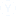what is the syllabus of PGT Mathematics

mathematics m.sc

• Anita
• 08 अक्तूबर
• 19864 दृश्य
• 2 उत्तर

PGT (Mathematics) of  syllabus

Sets
Relations & Functions
Principle of Mathematical Induction
Permutations & Combinations
Complex Numbers
Linear Inequalities
Binomial Theorem
Sequence and Serie
Elementary Number Theory
Matrices and Determinant
Two dimensional Geometry
Trigonometric Functions
Inverse Trigonometric Functions
Differential Calculus
Applications of Derivatives
Integral Calculus
Differential Equation
Vector
Three dimensional Geometry
Statistics
Probability
Linear Algebraअन्य संबंधित चर्चाएंमॉक परीक्षण अभ्यास के लिए
Army Welfare Education Society (AWES) PGT TGT PRT